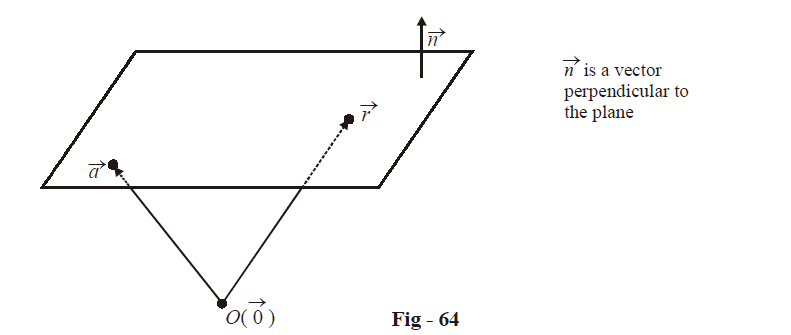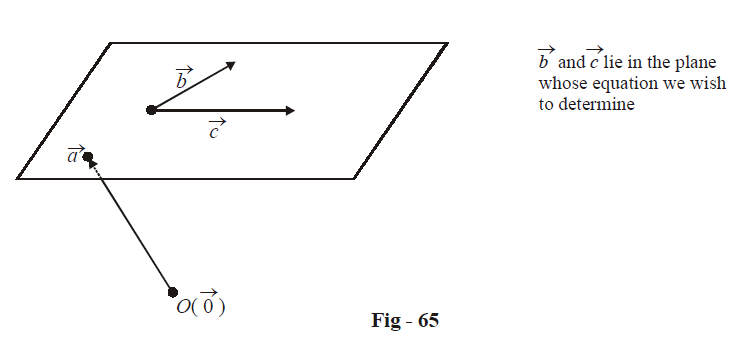# Vector Equations Of Planes

## VECTOR EQUATIONS OF A PLANE

Consider an arbitrary plane. How do you think that the equation of this plane can be specified? We need

(a)  either a point on the plane and the orientation of the plane (the orientation of the plane can be specified by the orientation of the normal of the plane).

(b)  or a point on the plane and two vectors coplanar with the plane.

Depending on whether we have the information as in (a) or as in (b), we have two different forms for the equation of the plane.

(a)  Let the plane be such that if passes through the point  $$\vec a$$ and  $$\vec n$$ is a vector perpendicular to the planeIt is evident that for any point  $$\vec r$$ lying on the plane, the vectors $$(\vec r - \vec a)$$ and  $$\vec n$$ are perpendicular. Thus,

\begin{align}&\qquad \; (\vec r - \vec a) \cdot \vec n = 0 \hfill \\\\& \Rightarrow \quad \boxed{\vec r \cdot \vec n = \vec a \cdot \vec n} \hfill \\ \end{align}

This is the required equation of the plane. Convince yourself that all (and only) points lying on the plane will satisfy this equation.

(b) Let the plane be such that it passes through the point  $$\vec a$$ and is parallel to the vectors $$\vec b$$ and $$\vec c$$ (in other words, is coplanar with vectors $$\vec b$$ and $$\vec c$$).It is assumed that $$\vec b$$ and $$\vec c$$ are non-collinear.Since $$\vec b$$ and $$\vec c$$ are non-collinear, any vector in the plane of $$\vec b$$ and $$\vec c$$ can be written as

$\lambda \vec b + \mu \vec c,\qquad\qquad\qquad where\,\lambda ,\,\mu \in \mathbb{R}$

Thus, any point lying in the plane can be written in the form

$\boxed{\vec r = \vec a + \lambda \vec b + \mu \vec c}\,\,\,\qquad for{\text{ }}some\,\,\lambda ,\,\mu \in \mathbb{R}$

This is the equation of the plane in parametric form. As we vary $$\lambda \,\,and\,\,\mu ,$$ we get different points lying in the plane.

To specify the equation of the plane in non-parametric form, note that for any point  $$\vec r$$ in the plane,$$(\vec r - \vec a)$$ lies in the plane of $$\vec b$$ and  $$\vec c$$ Thus, $$(\vec r - \vec a)$$ is perpendicular to $$\vec b \times \vec c:$$

\begin{align}&\quad\quad\; (\vec r - \vec a) \cdot (\vec b \times \vec c) = 0 \hfill \\\\& \Rightarrow \quad \vec r \cdot (\vec b \times \vec c) = \vec a \cdot (\vec b \times \vec c) \hfill \\\\& \Rightarrow \quad \boxed{\left[ {\vec r\,\,\,\,\,\vec b\,\,\,\,\,\vec c} \right] = \left[ {\vec a\,\,\,\,\,\vec b\,\,\,\,\,\vec c} \right]} \hfill \\ \end{align}

Convince yourself that all (and only) points $$\vec r$$ lying on the plane will satisfy this relation.

Vectors
grade 11 | Questions Set 1
Vectors
Vectors
grade 11 | Questions Set 2
Vectors